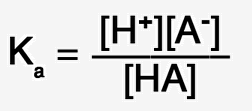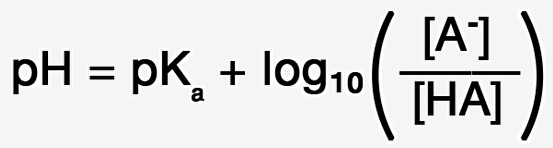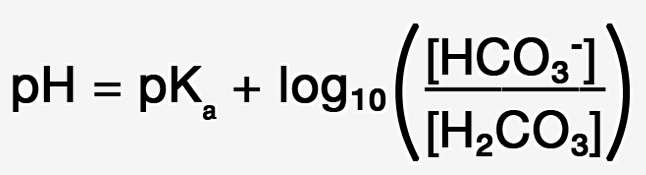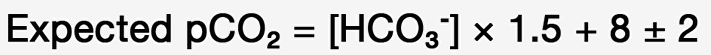If you’re like me, you learned and then promptly forgot the Henderson Hasselbalch equation (HH eq) in medical school.1 After all, in clinical rotations it was never invoked, and our patients seemed to have fared well without it. So why bring up the topic now?

Medicine is changing. The ubiquitous nature of computing allows a level of sophistication exponentially greater than before. To a large extent we’re freed from much of the onerous work of rote memorization. In the ideal, that should free us to be more thoughtful about the way we approach our work and to have a deeper understanding of health and disease. Going forward, medicine will become increasingly computational. With that in mind, I’ll make three points about the HH eq.

# 3 Important Points About the Henderson Hasselbalch Equation

1. The HH eq is a classic example of science at its best. There aren’t that many situations in medicine where understanding is achieved in a mathematically elegant and robust form. It should be astonishing to us that pH, pCO₂, and bicarbonate concentration are so intricately related and that we can perfectly quantitate that relationship.
2. We are capable of both understanding and manipulating the HH Eq. The logarithms are easy if you know just a few simple declarations. Log(A × B) is equal to logA + logB, and similarly log(A ÷ B) is the same as logA – logB. Finally, if logA = 4, then A  = 10⁴ .  That’s it. That’s all you need to know to derive the equation!
3. We can and should use it in the emergency department. One example of its use is for rapid estimation of pH and pCO₂ in patients with metabolic acidosis when the only data point available is a serum bicarbonate from a Chem 7. This requires the assumption of appropriate respiratory compensation.

# Derivation of the Henderson Hasselbalch EquationFigure 1. All acids in solution dissociate in a mathematically quantifiable way. Ka is the acid dissociation constant for that relationship.Figure 2. In medicine it is the convention to refer to acidity using pH instead of [H+], so the logarithm of both sides of the equation is taken.Figure 3. Remember that log(A × B) is equal to logA + logB, so we can rewrite the equation as shown aboveFigure 4. Since we want the equation to solve for pH, we’ll move the log[H+] to the left hand side of the equation and the log Ka to the right.Figure 5. By definition, pH means the negative log (base 10) of the hydrogen ion concentration. Similarly, pKₐ means the negative log of Ka, so we can rewrite the equation as shown above.Figure 6. We will be using the equation to scrutinize the bicarbonate buffer system, so carbonic acid and bicarbonate are substituted for HA and A respectively.Figure 7. Now we get to the really interesting part of the derivation. Carbon dioxide in solution forms carbonic acid, and there is a solubility constant described by Henry’s law that quantifies the relationship.

The solubility constant is 0.03, so we can substitute that into the equation to reach our final state. We’ll also substitute in the constant for pKₐ which is 6.1.Figure 8. The Henderson-Hasselbalch Equation as used in medicine

# The Equation In Clinical Practice

Consider a patient with a bicarbonate value of 12 mEq/L on a chemistry panel. A bicarbonate value of 24 is considered normal, with a range of 22 – 26. In most laboratories an autoanalyzer is used that measures total CO₂ content as an approximation of bicarbonate. This estimation will exceed the actual bicarbonate value by 1-2 mEq/L,2 so we may need to adjust for that. In this example we will use the actual value.

The low bicarbonate value indicates that an acidosis is present.

From our history and physical examination we will have a good sense whether our patient has some degree of respiratory compromise resulting in a respiratory acidosis. If it appears the patient has no respiratory compromise — and this could be confirmed by end tidal CO₂ monitoring — then we conclude there is a metabolic acidosis.

## Winters’ Equation

To estimate pH, we need one more value, the pCO₂. Assuming normal respiratory compensation, the pCO₂ is estimated using Winters’ equation,3 as follows:Figure 9. Winters’ equation

So our patient’s pCO₂ is 12 × 1.5 + 8 ± 2, which is 26 ± 2.

Entering those values into the HH eq gives a pH estimate of 7.27 (7.26 – 7.31). So what we’ve just accomplished is rapid estimation of pH starting with a bicarb value from a Chem 7. Or from a mathematical perspective, we used 2 equations to solve a three variable problem.

## The Decimal Digits Rule (aka “last 2 digits” rule)

As an alternative to the Winters’ equation, there’s another great way to estimate pCO₂ in metabolic acidosis using the “decimal digits” rule, sometimes referred to as the “last 2 digits” rule.4 The rule states that in patients with metabolic acidosis and appropriate respiratory compensation, the pCO₂ equals the decimal digits of the pH. The best way to understand this is with an example.

Imagine you are caring for a patient with DKA and you obtain a blood gas to estimate the severity of the illness. The results come back pH 7.27, pCO₂ 27, and bicarb 12.0. (The pO₂ is not relevant for the acid base analysis.) It’s helpful to know that the pH is 7.27, but equally important is whether there is adequate respiratory compensation for the acidosis. The “decimal digits” rule says that if the pH is 7.27, then the predicted pCO₂ in a patient with adequate respiratory compensation is 27. If your measured pCO₂ is close to predicted, then compensation is adequate. A pCO₂ much higher indicates inadequate respiratory compensation. This could be the case if your patient is extremely ill with respiratory muscle fatigue. A pCO₂ much lower indicates a primary respiratory alkalosis. Your patient is hyperventilating, and you should ask yourself why.

For the purposes of our work as emergency physicians, I’m arguing that we should work backwards using the “decimal digits” rule. Let’s start from scratch to create a lookup table that gives pH, pCO₂, and bicarb values that are compatible with the rule. How is that done? You guessed it. Using the Henderson Hasselbalch equation.

Start with pH 7.37 and a pCO₂ of 37 and calculate the predicted bicarb using HH eq. Then do the same thing for pH 7.36 and a pCO₂ of 36. Continue until you’ve reached a pCO₂ of 12, which is about the lower limit of respiratory compensation.

This technique works best if you actually use a pH with 3 decimal digits (i.e, 7.350 instead of 7.35) and a pCO₂ with 1 decimal digit (35.0 instead of 35). That way you can get bicarb results with three significant figures. OK, too much information. Take a look at the resulting table:

 pH pCO2 HCO3– 7.363 36.3 20.0 7.353 35.3 19.0 7.343 34.3 18.0 7.333 33.3 17.0 7.321 32.1 16.0 7.309 30.9 15.0 7.297 29.7 14.0 7.284 28.4 13.0 7.270 27.0 12.0 7.256 25.6 11.0 7.241 24.1 10.0 7.225 22.5 9.0 7.208 20.8 8.0 7.189 18.9 7.0 7.170 17.0 6.0 7.149 14.9 5.0 7.126 12.6 4.0 Table 1. Blood gas values derived from the “decimal digits” rule and the Henderson Hasselbalch Equation.

How do we use this table? The idea here is that when a Chem 7 result comes back with a significantly low bicarb, we know there is a metabolic acidosis. We use the table to predict pH and pCO₂ under the assumption that our patient has adequate respiratory compensation for the acidosis. So if my patient’s bicarb is 10, I expect to see a pCO₂ of 24 and a pH of 7.24.

# Case Studies

## Case 1

25 year old man, brought in for evaluation of a suspected suicide attempt by medication overdose. Chem 7 shows a bicarb of 13 and an anion gap of 20. If he has a pure metabolic acidosis with an appropriate degree of respiratory compensation, what would you expect his pCO₂ and pH to be?

Starting with the bicarb of 13, we can estimate the pCO₂ using Winters’ equation, so the expected pCO₂ is 13 × 1.5 + 8 ± 2 = 27.5 ± 2. Plugging those numbers into the Henderson Hasselbalch equation gives an expected pH of 7.28 (7.27 – 7.33). We would get the same results with the table generated by the “Decimal Digits” rule.

Here’s his actual blood gas: pH 7.50, pCO₂ 17. The pCO₂ is much lower than predicted indicating a respiratory alkalosis superimposed on the high anion gap metabolic acidosis. This is a classic finding in early aspirin overdose.

## Case 2

19 year old man found down unconscious, bicarb of 5, anion gap of 35. What are his expected pH and pCO₂?

Using the lookup table derived from the “Decimal Digits” rule, we expect a pH of 7.15 and a pCO₂ of 15. The actual values were pH of 7.00 and pCO₂ of 20. As you can see, the higher pCO₂ significantly lowers the pH. Any further compromise in his respiratory drive will push the pH into the dangerous sub 7 realm.

# To App or Not to App?

Should I use a look up table in a Paucis Verbis card, a mobile app, a web app, or something built into my EHR? How much time do you have? There probably are many situations in our practice where we just want an answer. That may seem shallow, but we don’t want to see or know the derivation if there’s not enough time or if we’re lacking the mental stamina at that particular moment. No problem. I’m like that. A lot. Use the tool that’s available and fast. But we can and should have the opportunity to take a deep dive from time to time, and that’s where a well designed app can make a difference if it has the ability to educate as well as spit out a result. Showing formulas and providing references is a minimum requirement. Particularly valuable is the use of animation. For now, be aware that you can accomplish the same end goal through a variety of means.

# Take Home Points

If you have a patient with a significantly low bicarb on a Chem 7, use these quick look-up tables to rapidly estimate pH and pCO₂ based on that single bicarbonate value. Alternatively, run the calculations from scratch, which is pretty awesome when you’re teaching residents and medical students at the bedside. Or use an app. Regardless of what method you choose, if you go on to get a blood gas, compare actual to predicted pCO₂.

If the pCO₂ agrees with predicted values, your patient has an appropriate respiratory compensation attenuating the acidosis. If the pCO₂ is too high, your patient also has a primary respiratory acidosis. The latter is a potentially dangerous scenario that may have an impact on how you manage the airway. On the other hand, if the pCO₂ is lower than expected, your patient has a primary respiratory alkalosis in addition to a metabolic acidosis. Think about aspirin overdose or other reasons why your patient might be hyperventilating.

And always remember to pay tribute to Henderson and Hasselbalch every time you interpret a blood gas.

Disclosures: Dr. Ruiz is the creator of the “Acid Base” app for the iPhone and “Likelihood” app for the iPad.

1.
Story D. Bench-to-bedside review: a brief history of clinical acid-base. Crit Care. 2004;8(4):253-258. [PubMed]
2.
Centor RM. Serum Total Carbon Dioxide. NCBI Bookshelf. https://www.ncbi.nlm.nih.gov/books/NBK308/. Published 1990.
3.
Albert M, Dell R, Winters R. Quantitative displacement of acid-base equilibrium in metabolic acidosis. Ann Intern Med. 1967;66(2):312-322. [PubMed]
4.
Fulop M. A guide for predicting arterial CO2 tension in metabolic acidosis. Am J Nephrol. 1997;17(5):421-424. [PubMed]#### @frankruizmd